StatLect

# Estimator

In the theory of parameter estimation, an estimator is a function that associates a parameter estimate to each possible sample we can observe.## Description

In a parameter estimation problem, we need to choose a parameter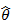from a parameter space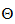, by using a sample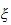(a set of observations from an unknown probability distribution).

The parameteris our best guess of the true and unknown parameter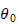, which is associated to (or describes) the probability distribution that generated the sample. The parameteris called an estimate of.

When the estimateis produced by using a predefined rule that associates a parameter estimateto each possible sample, we can writeas a function of: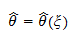The function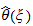is called an estimator.

## Estimators as random variables

The sample, before being observed, is regarded as a random variable, drawn from the distribution of interest. Therefore, the estimator, being a function of, is also regarded as a random variable.

After the sampleis observed, the realizationof the estimator is called an estimate of the true parameter. In other words, an estimate is a realization of an estimator.

## Examples

Commonly found examples of estimators are:

## How estimators are assessed

Different estimators of the same parameter are often compared by looking at their mean squared error, which is equal to the expected value of the squared difference between the estimator and the true value of the parameter. For an example of such comparisons, see the lecture on Ridge estimation.

## More details

More details about estimators can be found in the lecture entitled Point estimation, which discusses the concept of estimator and the main criteria used to evaluate estimators.